International
Tables for
Crystallography
Volume D
Physical properties of crystals
Edited by A. Authier

International Tables for Crystallography (2006). Vol. D, ch. 2.2, pp. 297-298

## Section 2.2.6. Space-group symmetry

K. Schwarza*

aInstitut für Materialchemie, Technische Universität Wien, Getreidemarkt 9/165-TC, A-1060 Vienna, Austria
Correspondence e-mail: kschwarz@theochem.tuwein.ac.at

### 2.2.6. Space-group symmetry

| top | pdf |

#### 2.2.6.1. Representations and bases of the space group

| top | pdf |

The effect of a space-group operation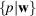on a Bloch function, labelled by, is to transform it into a Bloch function that corresponds to a vector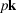,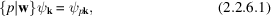which can be proven by using the multiplication rule of Seitz operators (2.2.3.12)and the definition of a Bloch state (2.2.4.17).

A special case is the inversion operator, which leads to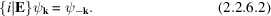The Bloch functions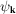and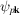, where p is any operation of the point group P, belong to the same basis for a representation of the space group G.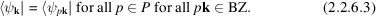The samecannot appear in two different bases, thus the two basesand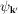are either identical or have noin common.

Irreducible representations of T are labelled by the N distinctvectors in the BZ, which separate in disjoint bases of G (with novector in common). If avector falls on the BZ edge, application of the point-group operation p can lead to an equivalent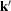vector that differs from the original by(a vector of the reciprocal lattice). The set of all mutually inequivalentvectors of(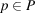) define the star of the k vector (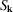) (see also Section 1.2.3.3of the present volume).

The set of all operations that leave avector invariant (or transform it into an equivalent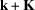) forms the groupof thevector. Application of q, an element of, to a Bloch function (Section 2.2.8) gives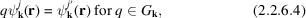where the band index j (described below) may change to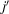. The Bloch factor stays constant under the operation of q and thus the periodic cell function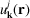must show this symmetry, namely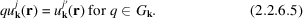For example, a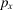-like orbital may be transformed into a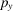-like orbital if the two are degenerate, as in a tetragonal lattice.

A star ofdetermines an irreducible basis, provided that the functions of the star are symmetrized with respect to the irreducible representation of the group ofvectors, which are called small representations. The basis functions for the irreducible representations are given according to Seitz (1937) by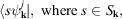written as a row vectorwith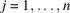, where n is the dimension of the irreducible representation ofwith the order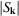. Such a basis consists offunctions and forms an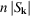-dimensional irreducible representation of the space group. The degeneracies of these representations come from the star of(not crucial for band calculations except for determining the weight of thevector) and the degeneracy from. The latter is essential for characterizing the energy bands and using the compatibility relations (Bouckaert et al., 1930; Bradley & Cracknell, 1972).

#### 2.2.6.2. Energy bands

| top | pdf |

Each irreducible representation of the space group, labelled by, denotes an energy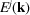, wherevaries quasi-continuously over the BZ and the superscript j numbers the band states. The quantization ofaccording to (2.2.4.13)and (2.2.4.15)can be done in arbitrary fine steps by choosing corresponding periodic boundary conditions (see Section 2.2.4.2). Sinceandbelong to the same Bloch state, the energy is periodic in reciprocal space: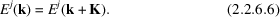Therefore it is sufficient to considervectors within the first BZ. For a given, two bands will not have the same energy unless there is a multidimensional small representation in the group ofor the bands belong to different irreducible representations and thus can have an accidental degeneracy. Consequently, this can not occur for a generalvector (without symmetry).

### References

Bouckaert, L. P., Smoluchowski, R. & Wigner, E. (1930). Theory of Brillouin zones and symmetry properties of wavefunctions in crystals. Phys. Rev. 50, 58–67.
Bradley, C. J. & Cracknell, A. P. (1972). The mathematical theory of symmetry in solids. Oxford: Clarendon Press.
Seitz, F. (1937). On the reduction of space groups. Ann. Math. 37, 17–28.